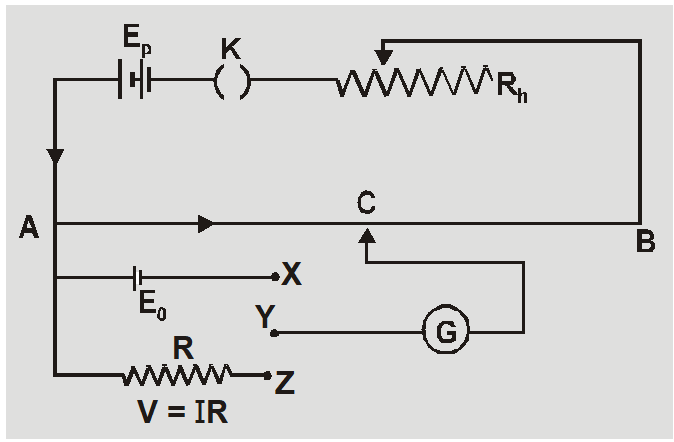Most Affordable JEE | NEET | 8,9,10 Preparation by Kota's Top IITian Doctor Faculties

# Uses of Potentiometer | Comparison of emf of two cells using Potentiometer || Class 12, JEE & NEETThe measuring instrument called a potentiometer is essentially a voltage divider used for measuring electric potential (voltage); the component is an implementation of the same principle, hence its name. Potentiometer are commonly used to control electrical devices such as volume controls on audio equipment. We will study here about Comparison of emf of two cells using Potentiometer, Determination of internal resistance of cell, Calibration of voltmeter, Calibration of ammeter, Measurement of small thermo emf.
7. ##### measurement of small thermo emf

PRECAUTIONS IN THE USE OF POTENTIOMETER

## Determination of unknown EMF or Potential Difference

1. Potential gradient is found by using a standard cell $x=E_{0} / \ell_{0}$
2. If unknown emf $\mathrm{E}_{1}$ is balanced at length $\ell_{1}$ then $E_{1}=x \ell_{1}=\left(\frac{E_{0}}{\ell_{0}}\right) \ell_{1}$3. If unknown potential difference V is balanced for length $\ell$ than $V=x \ell=\left(\frac{E_{0}}{\ell_{0}}\right) l$

## Precautions in use of Potentiometer

1. All high potential terminals of primary and secondary circuit should be connected at same point of Potentiometer.
2. The known emf of primary circuit must be greater than the unknown potential difference to be measured in secondary circuit.
3. The balancing length is always measured from the point of higher potential. In state of zero deflection no current flows in galvanometer circuit but it flows in primary circuit.